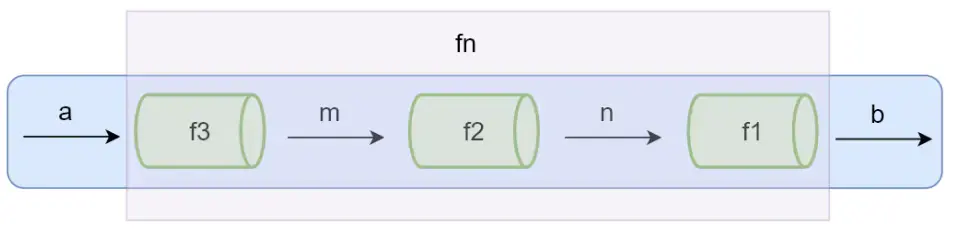# 1. 什么是函数式编程

1、下个路口左转

2、下个有红灯的路口右转

3、前进100米

4、在下个路口掉头

5、前进1500米

6、到达目的地出租车停车区

1、带我到XXX街。

1. 柯里化（curry）
2. 代码组合（compose）

# 2. 函数是一等公民

``````const add = (a, b) => a + b;

// 存入变量中
const compute = add; // 这里没有任何意义，在实际的开发中不推荐这么写

// 存入数组中
const list: any[] = [];
list.push(compute);``````

# 3. 纯函数

``````const xs = [1, 2, 3, 4, 5];

// 纯的
xs.slice(0, 3);
//=> [1,2,3]

xs.slice(0, 3);
//=> [1,2,3]

xs.slice(0, 3);
//=> [1,2,3]

// 不纯的
xs.splice(0, 3);
//=> [1,2,3]

xs.splice(0, 3);
//=> [4,5]

xs.splice(0, 3);
//=> []``````

``````// 不纯的
const minimum = 21;

const checkAge = function (age) {
return age >= minimum;
};

// 纯的
const checkAge = function (age) {
const minimum = 21;
return age >= minimum;
};``````

`Vue3`中，为了解决这个问题，引入了组合式api，它彻底实现了去`this`化。

# 4. 柯里化（curry）

``````const add = (x) => {
return (y) => {
return x + y;
};
};

increment(2);
// 3

// 12``````

`ramda`函数库中就提供了`curry`这样一个函数，你需要正常的书写函数，然后将函数传入`curry`这个函数中，它就会自动帮你进行`curry`化，是不是非常的方便？

``````import { curry } from "ramda"; // 引入ramda中的curry函数

const add = (x, y) => { // 正常编写函数
return x + y;
};

const newCurry = curry(add); // 进行柯里化
const increment = newCurry(1);

increment(2);
// 3

// 12``````

# 5. 代码组合（compose）`f3,f2,f1`这几个管道的统称，就叫做代码组合（compose）。

``````function compute(a, b, c, d) {
return (a + b) / c - d;
}``````

``````import { compose, curry } from "ramda";
// 两数相加
const add = curry((a: number, b: number): number => b + a);

// 两数相减
const divide = curry((a: number, b: number): number => b / a);``````

``````function compute(a, b, c, d) {
return (a + b) / c - d;
}

compute(3, 4, 5, 6); // -4.6``````

``````import { compose, curry } from "ramda";
// 两数相加
const add = curry((a: number, b: number): number => b + a);

// 两数相除
const divide = curry((a: number, b: number): number => b / a);

endValue(3); // -4.6``````

``````function compute(a, b, c, d, e) { // 改动
return (a + b) / c - d + e; // 改动
}

console.log(compute(3, 4, 5, 6, 10)); // 5.4``````

``````import { compose, curry } from "ramda";
// 两数相加
const add = curry((a: number, b: number): number => b + a);

// 两数相除
const divide = curry((a: number, b: number): number => b / a);

endValue(3); // 5.4``````

1. 代码简洁，开发快速
2. 接近自然语言，易于理解
3. 更方便的代码管理
4. 易于”并发编程”
5. 代码的热升级